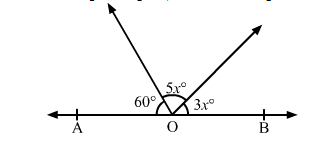# In the given figure, AOB is a straight line. The value of x is

Question:

In the given figure, AOB is a straight line. The value of x is(a) 12
(b) 15
(c) 20
(d) 25

Solution:

It is given that, AOB is a straight line.

∴ 60º + (5xº + 3xº) = 180º           (Linear pair)

⇒ 8xº = 180º − 60º = 120º

⇒ xº = 15º

Thus, the value of x is 15.

Hence, the correct answer is option (b).